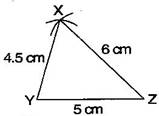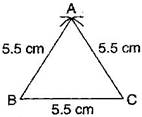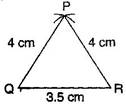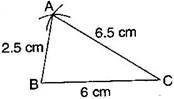### Practical Geometry - Solutions 2

CBSE Class –VII Mathematics
NCERT Solutions
Chapter 10 Practical Geometry (Ex. 10.2)

Question 1. Construct $\mathrm{\Delta }$XYZ in which XY = 4.5 cm, YZ = 5 cm and ZX = 6 cm.
Answer: To construct$\mathrm{\Delta }$XYZ, where XY = 4.5 cm, YZ = 5 cm and ZX = 6 cm.Steps of construction:
(a) Draw a line segment YZ = 5 cm.
(b) Taking Z as centre and radius 6 cm, draw an arc.
(c) Similarly, taking Y as centre and radius 4.5 cm, draw another arc which intersects first arc at point X.
(d) Join XY and XZ.
It is the required $\mathrm{\Delta }$XYZ.
Question 2. Construct an equilateral triangle of side 5.5 cm.
Answer: To construct: A $\mathrm{\Delta }$ABC where AB = BC = CA = 5.5 cmSteps of construction:
(a) Draw a line segment BC = 5.5 cm
(b) Taking points B and C as centers and radius 5.5 cm, draw arcs which intersect at point A.
(c) Join AB and AC.
It is the required $\mathrm{\Delta }$ABC.
Question 3. Draw $\mathrm{\Delta }$PQR with PQ = 4 cm, QR = 3.5 cm and PR = 4 cm. What type of triangle is this?
Answer: To construction$\mathrm{\Delta }$PQR, in which PQ = 4 cm, QR = 3.5 cm and PR = 4 cm.Steps of construction:
(a) Draw a line segment QR = 3.5 cm.
(b) Taking Q as centre and radius 4 cm, draw an arc.
(c) Similarly, taking R as centre and radius 4 cm, draw an another arc which intersects first arc at P.
(d) Join PQ and PR.
It is the required isosceles $\mathrm{\Delta }$PQR.
Question 4. Construct $\mathrm{\Delta }$ABC such that AB = 2.5 cm, BC = 6 cm and AC = 6.5 cm. Measure $\mathrm{\angle }$B.
Answer: To construct :$\mathrm{\Delta }$ABC in which AB = 2.5 cm, BC = 6 cm and AC = 6.5 cm.Steps of construction:
(a) Draw a line segment BC = 6 cm.
(b) Taking B as centre and radius 2.5 cm, draw an arc.
(c) Similarly, taking C as centre and radius 6.5 cm, draw another arc which intersects first arc at point A.
(d) Join AB and AC.
(e) Measure angle B with the help of protractor.
It is the required $\mathrm{\Delta }$ABC where $\mathrm{\angle }$B =